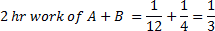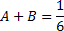## Time and Work For SBI PO Set – 05

1)  8 men and 4 women together can complete a piece of work in 6 days. The work done by a man in 1 day is double the work done by a woman in 1 day. If 8 men and 4 women started working and after 2 days 4 men left and 4 new women joined, in how many more days will the work be completed?

a)  5

b) 8

c) 6

d) 4

e)  9

a)  5

Explanation

1M = 2W

(8M+4W) × (6 days – 2 days)

= (4M+8W) × x days

(8× 2W + 4W) × (6 – 2) days

= (4× 2W + 8W) × x days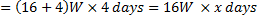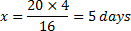2) A, B and C have to type 506 pages to finish an assignment. A can type a page in 12 minutes, B in 15 minutes and C in 24 minutes. If they divide the task into three parts so that all three of them spend equal amount of time in typing, what is the number of pages that B should type?

a)   172

b)  176

c) 154

d)  168

e)  174

b)  176

Explanation

Ratio of their efficiency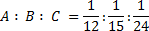= 10 : 8 : 5

Total number of pages = 506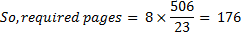3)  Pipe A is opened to fill a cistern. After 2 hours B is opened to fill the remaining tank. Had A left the tank filled after 3 hours, B would have filled the tank in 6 hours. In how many hours B alone can fill the tank?

a)   15 hours

b)   6 hours

c) 10 hours

d)  5 hours

e)  8 hours

a)   15 hours

Explanation

From the data given, we can equate

2x+9y=3x+6y

X=3y

2x+9y=(2×3y)+9y=6y+9y=15y

Y=1/15

4) A can do the work in 10 days, B can do the same work in 15 days and C can do the same work in 20 days. A started working alone. B joined after 2 days. C joined with both A and B one day after B joined. Find the total number of days required to finish the work?

a)    5    7/11

b)  5   9/13

c)  5  11/13

d)   5 12/13

e) 5    9/13

d)   5 12/13

Explanation

LCM of 10,15,20 =60

So, total wok to be done is 60 units.

A does 6 units per day

B does 4 units per day

C does 3 units per day

A does the work in 2 days=12 units

Remaining 48 units

A+B in 1 day= 10 units

Remaining=38 units

A+B+C=38/(6+4+3)=38/13=2   12/13

Total work done in 2+1+38/13=5  12/13

5) A, B, and C can complete a piece of work in 15, 20 and 30 days respectively. All started working together, but A left after some days. If B and C completed the remaining work in 3 more days, then how much work is completed by A?

a)   1/4

b)  1/3

c)  1/6

d) 1/8

e)  1/5

b)  1/3

Explanation

If A do work for x days, then B and C for (x+3) days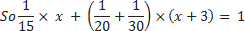Solve, x = 5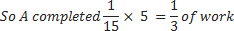6) There are two taps to fill a tank while a third to empty it. When the third tap is closed, they can fill the tank in 10 minutes and 12 minutes, respectively. If all the three taps be opened, the tank is filled in 15 minutes. If the first two taps are closed, in what time can the third tap empty the tank when it is full?

a)   8 min and 34 sec

b)  9 min and 32 sec

c)  7 min

d)  6 min

e) None of these

a)   8 min and 34 sec

7) Vasu can do a piece of work in 24 days, Seethu can do the same work in 16 days, and Sunil can do the same job in 4/5th time required by both Vasu and Seethu. Vasu and Seethu work together for 6 days, then Sunil completes the job. How many days did Sunil work?

a)   21/25

b)  4

c)  2  22/25

d)  2

e) 2  24/25

c)  2  22/25

8) Hari and Vijay can together finish a work in 30 days. They worked together for 20 days and then Vijay left. After another 20 days, hari finished the remaining work. In how many days hari alone can finish the work?

a)  45

b)  60

c)  35

d)  50

e)  65

b)  60

9) Dinesh does 80% of a work in 20 days. He then calls in Gokul and they together finish the remaining work in 3 days. How long Gokul alone would take to do the whole work?

a)   39

b)  37

c)  37 ½ days

d)  40 days

e)  39 ½ days

c)  37 ½ days

10) Two pipes A and B can fill a tank in 12 hours and 4 hours respectively. If they are opened on alternate hours and if pipe A is opened first, in how many hours will the tank be full?

a)  1.4 hours

b) 5

c)  2

d)  6

e)  8

d)  6

Explanation